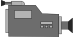Factoring Quadratics (Using Diamond Problems)Factoring Quadratics (Without Using Diamond Problems)## 1. Factoring Trinomials (Using Diamond Problems):

• Setup:
• Record information:
• Use the information for factoring.

## 2. Factoring Trinomials (Without Using Diamond Problems):

• Set up:
• Recording the sum:
• Figuring out the factors: